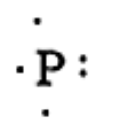QuestionAnswers

# How would you draw a Lewis structure for an atom that has an electronic configuration $1{s^2}2{s^2}2{p^6}3{s^2}3{p^3}$Verified
92.4k+ views
Hint:In order to draw a Lewis dot structure for a given electronic configuration $1{s^2}2{s^2}2{p^6}3{s^2}3{p^3}$, we must first find the atomic number of the atom. This can be done by counting the electrons present in the electronic configuration.